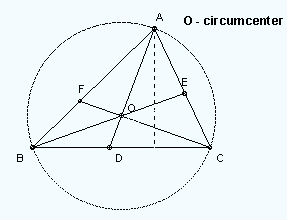Math Calculator

#### Circumcenter of Triangle, Bisector

The point where the perpendicular bisectors of triangle meet is known as circumcenter. Calculate the circumcenter of a triangle from the known values of co-ordinates.

#### Calculator

 Enter the coordinates of a triangle: X Y A: B: C: Triangle Circumcenter:

#### Definition

The point where the perpendicular bisectors of a triangle meet is called the Circumcenter. It lies inside for an acute, outside for an obtuse and at the center of the hypotenuse for the right triangle. Perpendicular bisectors are nothing but the line or a ray which cuts another line segment into two equal parts at 90 degree. Hypotenuse is the longest side of the right-angled triangle, i.e., the side opposite the right angle.

#### Example

In the below example, O is the Circumcenter.#### Method to calculate the circumcenter of a triangle

Let the points of the sides be A(5,7), B(6,6) and C(2,-2). Consider the points of the sides to be x1,y1 and x2,y2 respectively. We need to find the equation of the perpendicular bisectors to find the points of the Circumcenter.

`Step 1 :`

Lets calculate the midpoint of the sides AB, BC and CA which is the average of the x and y co-ordinates. Midpoint of a line in the triangle = x1+x2/2, y1+y2/2 Midpoint of AB = 5+6/2, 7+6/2 = (11/2, 13/2) Midpoint of BC = 6+2/2, 6-2/2 = (4, 2 Midpoint of CA = 2+5/2, -2+7/2 = (7/2, 5/2)

`Step 2 :`

Next, we need to find the slope of the sides AB, BC and CA using the formula y2-y1/x2-x1. Kindly note that the slope is represented by the letter 'm'. Slope of AB (m) = 6-7/6-5 = -1. Slope of BC (m) = -2-6/2-6 = 2. Slope of CA (m) = 7+2/5-2 = 3.

`Step 3 :`

Now, lets calculate the slope of the perpendicular bisector of the lines AB, BC and CA. The slope of the perpendicular bisector = -1/slope of the line. Slope of the perpendicular bisector of AB = -1/-1 = 1 Slope of the perpendicular bisector of BC = -1/2 Slope of the perpendicular bisector of CA = -1/3

`Step 4 :`

Once we find the slope of the perpendicular lines, we have to find the equation of the perpendicular bisectors with the slope and the midpoints. Lets find the equation of the perpendicular bisector of AB with midpoints (11/2,13/2) and the slope 1. Formula to find the circumcenter equation y-y1 = m(x-x1) y-13/2 = 1(x-11/2) By solving the above, we get the equation -x + y = 1 -------------1 Similarly, we have to find the equation of the perpendicular bisectors of the lines BE and CF. For BC with midpoints (4,2) and slope -1/2 y-2 = -1/2(x-4) By solving the above, we get the equation x + 2y = 8 -------------2 For CA with midpoints (7/2,5/2) and slope -1/3 y-5/2 = -1/3(x-7/2) By solving the above, we get the equation x + 3y = 11 ------------3

`Step 5 :`

Find the value of x and y by solving any 2 of the above 3 equations. In this example, the values of x any y are (2,3) which are the coordinates of the Circumcenter (o).